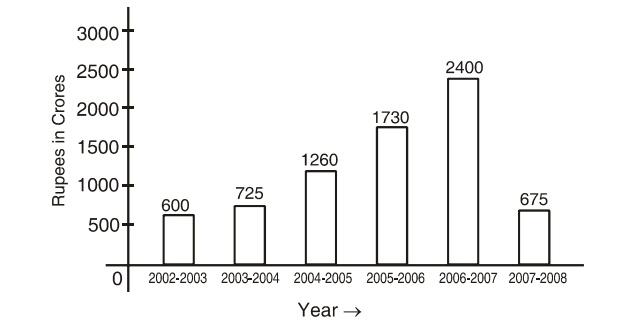## Bar graph

#### Data Interpretation

Direction: The following bar chart shows the amount accrued from additional taxation by the government during certain successive years. Study the chart carefully and answer the questions that follow:1. In between which of the following two successive finacial years increase in taxation was at the highest level?

1. Between the financial years 2005-06 and 2006-07, maximum incresase of Rs. 670 cores had been registered in taxation.

##### Correct Option: B

Between the financial years 2005-06 and 2006-07, maximum incresase of Rs. 670 cores had been registered in taxation.

1. Total increase in taxation was how much percent between the years 2004-05 and 2005-06?

1. Percentage of total increase = (1730 - 1260)/ 1260 x 100

##### Correct Option: A

Percentage of total increase = (1730 - 1260)/ 1260 x 100 = 470 x 100/ 1260 = 37.3%

1. Percentage decrease in taxation in between the years 2006-7 and 2007-08 was:

1. Percentage of total decrease in taxation between the years 2006-07 and 2007-08.

##### Correct Option: A

Percentage of total decrease in taxation between the years 2006-07 and 2007-08
= (2400 - 675/ 2400 x 100 = 1725 x 100/ 2400 = 71.8%

1. Between the years 2005-06 and 2006-07 the taxation was increased by:

1. Increase in taxation in between the financial years 2005-06 and 2006-07.

##### Correct Option: A

Increase in taxation in between the financial years 2005-06 and 2006-07
= Rs. (2400 - 1730) = RS. 670 crores

1. What is the average amount of taxation during all these years?

1. Average of the total taxation during the years = total taxation for all the years / 6.

##### Correct Option: C

Average of the total taxation during the years = total taxation for all the years / 6.
Average of the total taxation during the years = 600 + 725 + 1260 + 1730 + 2400 + 625 = 7390/6 = 1231.7 crores